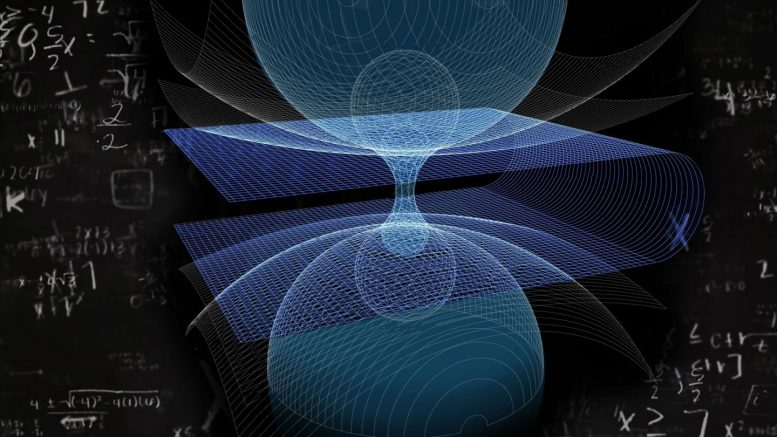# Physicist Details the Shape of a Symmetrical WormholeRUDN physicist demonstrated how to describe the shape of any symmetrical wormhole — a black hole that theoretically can be a kind of a portal between any two points in space and time. Federico.ciccarese/Wikimedia Commons/Allen Dressen

A RUDN physicist demonstrated how to describe the shape of any symmetrical wormhole – a black hole that theoretically can be a kind of a portal between any two points in space and time – based on its wave spectrum. The research would help understand the physics of wormholes and better identify their physical characteristics. The article of the scientist was published in the Physics Letters B journal.

Modern concepts of the Universe provide for the existence of wormholes – unusual curvatures in space and time. One can imagine a wormhole as a black hole through which one can see a distant point of the Universe in four dimensions. Astrophysicists are still unable to precisely determine the shape and sizes of black holes, let alone the wormholes that exist only in theories. A RUDN physicist demonstrated that the shape of a wormhole still can be calculated based on observable physical characteristics.

In practice, one can observe only indirect properties of wormholes, such as red shift – a downward shift in the frequency of gravitational waves in the course of moving away from an object. A researcher from RUDN Institute of Gravitation and Cosmology used quantum mechanical and geometrical assumptions and showed that the shape and mass of a wormhole can be calculated based on the red shift value and the range of gravitational waves in high frequencies.

Today the scientists deal with direct tasks: they take the geometry of a compact object, find out its range (the set of frequencies at which a wormhole emits gravitational waves), and then compare the data with experimental results. After that they decide whether the observed values are similar to theoretically predicted ones. The author of the work suggested a solution to an opposite problem: he managed to determine the shape of an object based on its visible spectrum.

The physicist took a mathematical model of a spherically symmetrical Morris-Thorne wormhole – a type of black holes that not only unites two points in space and time but theoretically provide for movements between them. Then he applied an existing mathematical model to describe the bottleneck of the wormhole – the narrowest place between its entrance and exit. First, he mathematically described how the shape of any symmetrical wormhole can be determined based in its wave range, and solved the so-called opposite problem in general terms. Then, using quantum mechanical approximation, he established an equation to calculate a geometrical shape for a particular case – a wormhole.

“In general terms a quantum mechanical approach leads to many solutions for the geometry of a wormhole. Our work can be expanded in several ways. First of all, to avoid long formulae, we only considered electromagnetic fields. In our future work we can study other fields under the same approach. Our results may be applied to rotating wormholes as well, provided they are symmetrical enough,” says Roman Konoplya, the author of the work, and a research assistant at RUDN Institute of Gravitation and Cosmology.

Publication: “How to tell the shape of a wormhole by its quasinormal modes” R.A.Konoplya, 17 July 2018, Physics Letters B.
DOI: 10.1016/j.physletb.2018.07.025

#### 1 Commenton "Physicist Details the Shape of a Symmetrical Wormhole"

1.David Raften | October 19, 2018 at 4:44 am | Reply

Pardon my ignorance, I am not a physicist. I had thought “Red Shift” is due to objects moving away from us, while a “Reddening,” is photons losing energy due to fighting gravity. Red Shift moves the spectrum lines, while reddening just changes the color. So how can using red shift be used to determine shape and mass? Do you mean “Reddening”?